• 非常适用于初学者，有详细算法实现过程，用于分类的例子，用于回归的例子，可以帮你深入理解随机森林算法哦~
• ## 随机森林算法原理

万次阅读 2019-02-22 12:33:54
决策树与随机森林学习笔记 为什么要用-p*log('p)这个公式? 这个问题先放下，看看下面这些问题 假设我们用了一个只能装8个分子的盒子装了8个分子，当容器中存在空隙时分子会任意乱飞，系统的熵值本来就是表示...


文章目录
熵值法介绍熵值法公式的理解
决策树的原理及Python实现bagging的原理最小二乘回归树算法实现一个简单的最小二乘预测器线性回归器简单示例

熵值法介绍

熵定义：熵值法是计算指标权重的经典算法之一，它是指用来判断某个指标的离散程度的数学方法。其和化学里面的概念是相同的，指物体内部的混乱程度。混乱程度（纯度）低则熵值小，反之！ 查看了很多文献，好像对熵值的解读都很高深，现在说下自己的理解

熵值法公式的理解
熵值公式链接 决策树与随机森林学习笔记

为什么要用-p*log('p)这个公式? 这个问题先放下，看看下面这些问题 假设我们用了一个只能装8个分子的盒子装了8个分子，当容器中存在空隙时分子会任意乱飞，系统的熵值本来就是表示物体内部的混乱程度的，这时我们可以用公式可以求出系统的总熵值为0（ 0和log函数相乘无限趋近于0时值为0），同理当盒子中没有分子时总熵值也为0 这时我们考虑两个系统，一个容积为4个分子的器皿装了2个分子，和一个容积为8个分子的器皿装了4个分子，他们的熵值是不是相同，当然一样。他们的总熵值都为1，也就是他们都处于最极端混乱的状态。 注：容积为8的器皿装5个分子和装3个分子，他们的混乱状态相同 同一个系统中，熵值是满足相加性的，熵值只是一个相对属性，不要把不同系统的熵值搞混

问什么要用-p*log('p)这公式？因为他满足以下条件，自己可以试着去掉一些参数看看它满不满足，熵值公式必须满足了系统以下几个特征： 1：连续性 该量度应连续，概率值小幅变化只能引起熵的微小变化 2：对称性 符号xi重新排序后，该量度应不变，这也是我们可以归一化、标准化矩阵参数的原因，因为无论怎么变，行向量的混乱程度的都不会变 3：可加性 熵的量与该过程如何被划分无关 4：极值性 当所有符号有同等机会出现的情况下，熵达到最大值（所有可能的事件同等概率时不确定性最高）

熵值算法的例子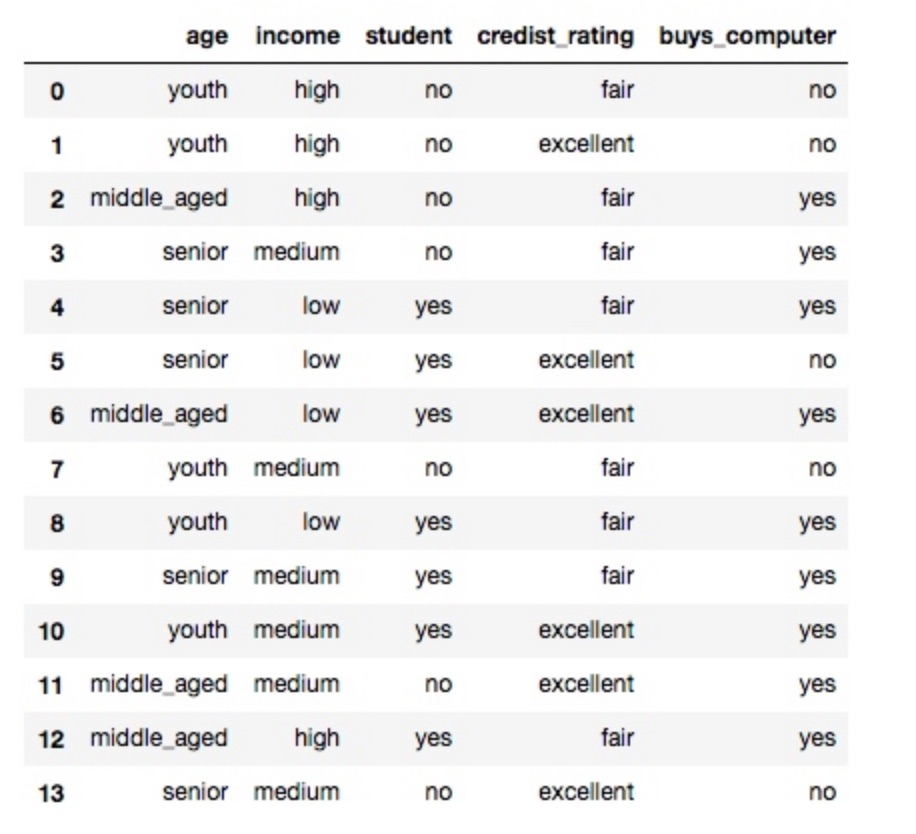上面链接中的大神已经为我们做了详细解释，我简单对熵值算法坐下解释： 我们对比整个表的第一列，可以看出第一列有3个属性：
系统一开始的熵值: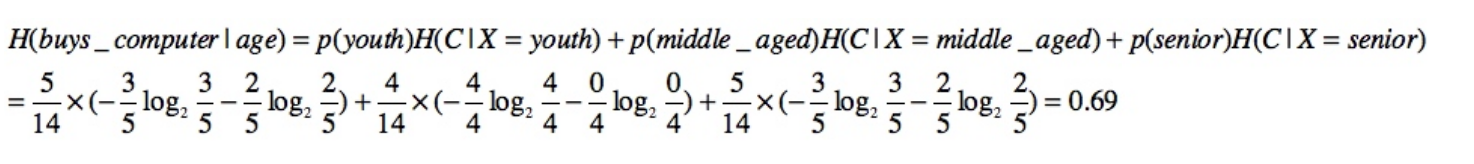最后合并成一个系统后的熵值：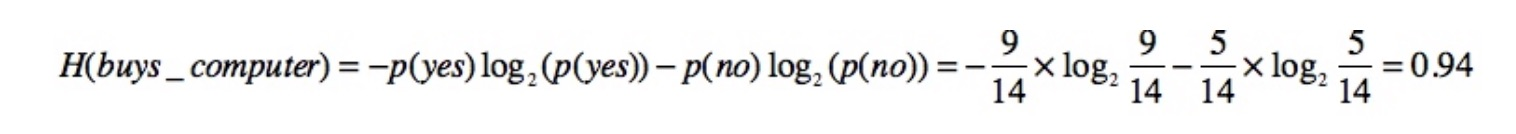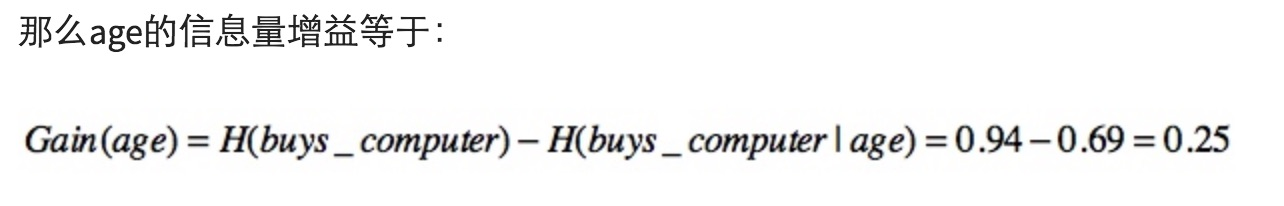其余列的增益可以从链接文档中查看。 简而言之系统的状态变化越大，熵值变化量越大，代表列越复杂，它所包含的信息量也越多，它对输出结果的影响也越大，它在决策树中的层级也就越高。
决策树的原理及Python实现
决策树的原理和实现链接1 决策树的原理和实现链接2
bagging的原理
集成学习有两个流派，一个是boosting派系，它的特点是各个弱学习器之间有依赖关系。另一种是bagging流派，它的特点是各个弱学习器之间没有依赖关系，可以并行拟合从下图可以看出，Bagging的弱学习器之间确没有boosting那样的联系。它的特点在于“随机采样”。那么什么是随机采样？
随机采样(bootsrap)就是从我们的训练集里面采集固定个数的样本，但是每采集一个样本后，都将样本放回。也就是说，之前采集到的样本在放回后有可能继续被采集到。对于我们的Bagging算法，一般会随机采集和训练集样本数m一样个数的样本。这样得到的采样集和训练集样本的个数相同，但是样本内容不同。如果我们对有m个样本训练集做T次的随机采样，则由于随机性，T个采样集各不相同。
注意到这和GBDT的子采样是不同的。GBDT的子采样是无放回采样，而Bagging的子采样是放回采样。
对于一个样本，它在某一次含m个样本的训练集的随机采样中，每次被采集到的概率是1/m。不被采集到的概率为1/m−1。如果m次采样都没有被采集中的概率是(1−1/m)^m 　　 当m→∞时，(1−1/m)^m→1e≃0.368。也就是说，在bagging的每轮随机采样中，训练集中大约有36.8%的数据没有被采样集采集中。
对于这部分大约36.8%的没有被采样到的数据，我们常常称之为袋外数据(Out Of Bag, 简称OOB)。这些数据没有参与训练集模型的拟合，因此可以用来检测模型的泛化能力。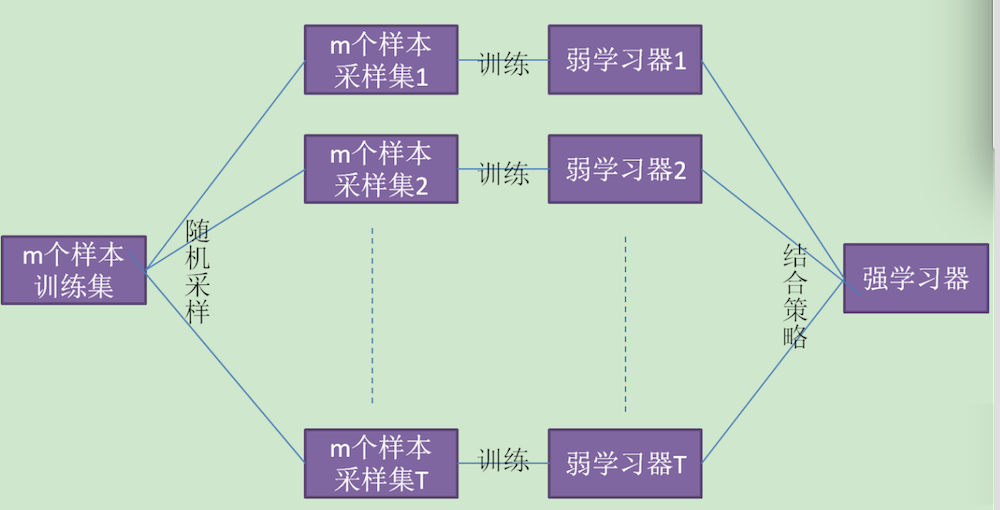最小二乘回归树算法实现
不想看这一堆公式就跳过去看下面内容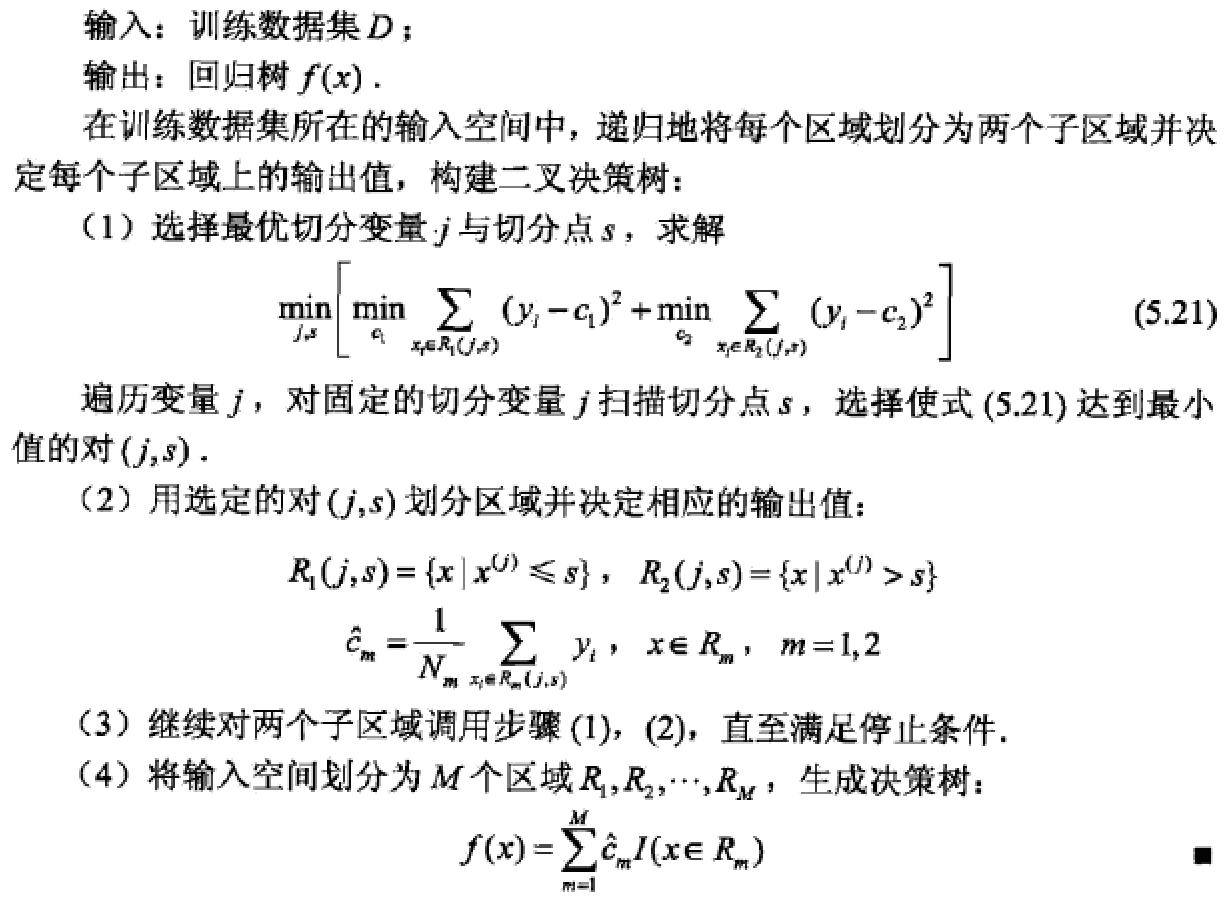一个简单的最小二乘预测器
我们拿上面图一的简单方程说起，我们构造2组数据： 两个方程参数分别接近（1，2，3，4，5），（1，2，2，4，5） 我们可以自己动手加下，只有最后一组数据有些许偏差（16—>15, 15---->14)
	X1 = np.array([[-2,-1,0,1,2],[-1,0,1,2,3],[1,1,1,0,0],[1,1,0,1,0],[1,0,0,0,1],[1,1,1,1,1]])
X2 = np.array([[1,0,0,2,1],[-1,0,2,1,1],[-3,1,2,0,0],[-4,2,0,1,1],[1,1,0,0,0],[1,1,1,1,1]])
Y1 = np.array([,,,,,])
Y2 = np.array([,,,,,])
X = np.vstack((X1,X2))
Y = np.vstack((Y1,Y2))

这时我们可以得到两个2维（我们用几个下标可以把X表示出来，可以用X.ndim算出) 简单方程，2*6组数据5个系数：
class Least_square:
def __init__(self):
self.param = np.array([])
self.Y = np.array([])

def fit(self, X, Y):
X_T = X.transpose()
self.param = np.dot(np.dot(np.linalg.inv(np.dot(X_T,X)),X_T),Y)
self.Y = Y
return self.param

def predict(self,X):
result = np.dot(X,self.param)
var = np.var(self.Y - result)
return result, var

least_instance = Least_square()
param = least_instance.fit(X,Y)
result,var = least_instance.predict(X)

线性回归器简单示例
from sklearn.linear_model import LinearRegression
regression = LinearRegression()
param = regression.fit(X, Y)
score = regression.score(X,Y)
result = regression.predict(X)


展开全文• 集成算法 ensemble 通过在数据集上构建多个模型，集成所有模型的建模结果，得到一个综合的结果，以此来获得比单个 ...装袋法的代表模型就是随机森林 提升法boosting: 基评估器是相关的，是按顺序一一...
集成算法 ensemble
通过在数据集上构建多个模型，集成所有模型的建模结果，得到一个综合的结果，以此来获得比单个
模型更好的分类或回归表现

装袋法bagging：
构建多个相互独立的基评估器（base estimator）,然后对其预测进行平均或多数表决原则来决定
集成评估器的结果。装袋法的代表模型就是随机森林
提升法boosting:
基评估器是相关的，是按顺序一一构建的。其核心思想是结合弱评估器的力量一次次对难以评估的样本

装袋法和提升法对比
随机森林参数
控制基评估器参数：
与决策树类似
n_estimators：
决策树的数量，越大，模型的效果往往越好。但是相应的，任何模型都有决策边界，n_estimators达到
一定的程度之后，随机森林的精确性往往不再上升或开始波动

%matplotlib inline
from sklearn.tree import DecisionTreeClassifier
from sklearn.ensemble import RandomForestClassifier

wine = load_wine()

决策树与随机深林的对比
from sklearn.model_selection import train_test_split
Xtrain, Xtest, Ytrain, Ytest = train_test_split(wine.data,wine.target,test_size=0.3)

clf = DecisionTreeClassifier(random_state=0)
rfc = RandomForestClassifier(random_state=0)
clf = clf.fit(Xtrain,Ytrain)
rfc = rfc.fit(Xtrain,Ytrain)
score_c = clf.score(Xtest,Ytest)
score_r = rfc.score(Xtest,Ytest)

print("Single Tree:{}".format(score_c)
,"Random Forest:{}".format(score_r)
)

Single Tree:0.9444444444444444 Random Forest:1.0

#交叉验证情况下
from sklearn.model_selection import cross_val_score
import matplotlib.pyplot as plt

rfc = RandomForestClassifier(n_estimators=25)
rfc_s = cross_val_score(rfc,wine.data,wine.target,cv=10)

clf = DecisionTreeClassifier()
clf_s = cross_val_score(clf,wine.data,wine.target,cv=10)

plt.plot(range(1,11),rfc_s,label = "RandomForest")
plt.plot(range(1,11),clf_s,label = "Decision Tree")
plt.legend()
plt.show()

[外链图片转存失败,源站可能有防盗链机制,建议将图片保存下来直接上传(img-X1SsirVY-1587530490929)(output_12_0.png)]
# 多次交叉验证
rfc_l = []
clf_l = []

for i in range(10):
rfc = RandomForestClassifier(n_estimators=25)
rfc_s = cross_val_score(rfc,wine.data,wine.target,cv=10).mean()
rfc_l.append(rfc_s)
clf = DecisionTreeClassifier()
clf_s = cross_val_score(clf,wine.data,wine.target,cv=10).mean()
clf_l.append(clf_s)

plt.plot(range(1,11),rfc_l,label = "Random Forest")
plt.plot(range(1,11),clf_l,label = "Decision Tree")
plt.legend()
plt.show()

#是否有注意到，单个决策树的波动轨迹和随机森林一致？
#再次验证了我们之前提到的，单个决策树的准确率越高，随机森林的准确率也会越高

[外链图片转存失败,源站可能有防盗链机制,建议将图片保存下来直接上传(img-rGAG65xY-1587530490936)(output_14_0.png)]
n_estimator 参数曲线
#####【TIME WARNING: 2mins 30 seconds】#####
superpa = []
for i in range(200):
rfc = RandomForestClassifier(n_estimators=i+1,n_jobs=-1)
rfc_s = cross_val_score(rfc,wine.data,wine.target,cv=10).mean()
superpa.append(rfc_s)
print(max(superpa),superpa.index(max(superpa))+1)#打印出：最高精确度取值，max(superpa))+1指的是森林数目的数量n_estimators
plt.figure(figsize=[20,5])
plt.plot(range(1,201),superpa)
plt.show()

0.9888888888888889 32

[外链图片转存失败,源站可能有防盗链机制,建议将图片保存下来直接上传(img-5ATU1OOh-1587530490942)(output_16_1.png)]
随机森林为什么准确率高？
随机森林的本质是一种装袋集成算法（bagging），装袋集成算法是对基评估器的预测结果进行平均
或用多数表决原则来决定集成评估器的结果。

在刚才的红酒例子中，我们建立了25棵树，对任何一个样本而言，平均或多数表决原则下，当且仅当
有13棵以上的树判断错误的时候，随机森林才会判断错误。单独一棵决策树对红酒数据集的分类准确
率在0.85上下浮动，假设一棵树判断错误的可能性为0.2，那13棵树以上都判断错误的可能性是

import numpy as np
from scipy.special import comb

np.array([comb(25,i)*(0.2**i)*((1-0.2)**(25-i)) for i in range(13,26)]).sum()


0.00036904803455582827

可以看到：远小于一棵树的错误率。同时也注意到，如果随机森林中每棵树都是一样的话，
那么一棵树判断错误，则整个森林就判读错误，也就和决策树没有区别。

所以随机森林的基分类器是相互独立的，互不相同的

怎样生成不同的树 ------双重随机性
参数：random_state 控制森林生成的模式
rfc = RandomForestClassifier(n_estimators=20,random_state=2)
rfc = rfc.fit(Xtrain, Ytrain)

#重要属性：estimators_ 存储所有树信息的列表
#查看所有树的random_state
for i in range(len(rfc.estimators_)):
print(rfc.estimators_[i].random_state)

2056096140
1078729000
602442957
1514174439
1458549053
904046564
1214918618
655921571
139775012
293793817
864952371
2116213231
963777025
861270369
1156416813
2032972974
583060530
1909517413
1341241096
2058549495
533227000
1803803348
2056406370
1190856758
132094869

当random_state固定时，随机森林中生成是一组固定的树，但每棵树依然是不一致的，这是用
”随机挑选特征进行分枝“的方法得到的随机性。并且我们可以证明，当这种随机性越大的时
候，袋装法的效果一般会越来越好。

但这种做法的局限性是很强的，当我们需要成千上万棵树的时候，数据不一定能够提供成千上
万的特征来让我们构筑尽量多尽量不同的树

参数：bootstrap &oob_score 有放回的随机抽样
要让基分类器尽量都不一样，一种很容易理解的方法是使用不同的训练集来进行训练，而袋装
法正是通过有放回的随机抽样技术来形成不同的训练数据。

参数bootstrap默认为True，即默认使用有放回的随机抽样

有放回随机抽样：
在一个含有n个样本的原始训练集中，我们进行随机采样，每次采样一个样本，并在抽取下
一个样本之前将该样本放回原始训练集，也就是说下次采样时这个样本依然可能被采集到，
这样采集n次，最终得到一个和原始训练集一样大的，n个样本组成的自助集

然而,由于是有放回，一些样本可能在同一个自助集中出现多次，而其他一些却可能被忽略，
因为每一个样本被抽到某个自助集中的概率为：


$1-(1-\frac{1}{n})^n$

$\displaystyle\lim_{n\to\infty}(1-(1-\frac{1}{n})^n)=(1-\frac{1}{e})=0.632$

一般来说，一个自助集大约平均会包含63%的原始数据。会有约37%的训练数据被浪费掉，没有
参与建模，这些数据被称为袋外数据(out of bag data，简写为oob)。除了我们最开始就划分好
的测试集之外，这些数据也可以被用来作为集成算法的测试集

也就是说，在使用随机森林时，我们可以不划分测试集和训练集，只需要用袋外数据来测试我们
的模型即可


rfc = RandomForestClassifier(n_estimators=25,oob_score=True) #默认为False
rfc = rfc.fit(wine.data,wine.target)

#重要属性 oob_score_ 使用袋外数据的模型评分
rfc.oob_score_

0.9719101123595506

当然，这也不是绝对的，当n和n_estimators都不够大的时候，很可能就没有数据掉落在袋外
，自然也就无法使用oob数据来测试模型了

5个重要接口
fit:
训练模型，输入训练集特征和标签

score:
返回分类准确率，可以是测试集也可以使训练集。注意，指标是不能修改的，如果想使用其他
衡量指标，则不用score，使用交叉验证。

    apply:
返回每个样本所在叶子节点索引,可以是测试集也可以使训练集

    predict:
返回每个样本的分类结果,可以是测试集也可以使训练集

    predict_proba:
返回每个样本的分类到某一类的概率,可以是测试集也可以使训练集

rfc = RandomForestClassifier(n_estimators=25,random_state=20)
rfc = rfc.fit(Xtrain, Ytrain)
rfc.score(Xtest,Ytest)

rfc.apply(Xtest)

array([[10, 16, 16, ...,  8,  4,  6],
[26, 18, 20, ..., 20, 14, 20],
[10, 16, 16, ...,  8,  4,  2],
...,
[26, 22, 20, ..., 20, 14, 15],
[15,  4,  4, ..., 10, 11, 14],
[19, 16, 16, ..., 19,  7,  2]], dtype=int64)

rfc.predict(Xtest)

array([2, 0, 2, 1, 0, 1, 0, 1, 0, 0, 2, 0, 1, 1, 0, 1, 0, 0, 1, 1, 2, 1,
0, 0, 0, 1, 0, 1, 2, 0, 0, 2, 0, 0, 1, 1, 2, 0, 1, 2, 2, 1, 2, 2,
2, 1, 0, 1, 1, 0, 0, 0, 1, 2])

rfc.predict_proba(Xtest)

array([[0.  , 0.  , 1.  ],
[1.  , 0.  , 0.  ],
[0.  , 0.  , 1.  ],
[0.  , 1.  , 0.  ],
[0.84, 0.16, 0.  ],
[0.  , 0.96, 0.04],
[0.88, 0.12, 0.  ],
[0.12, 0.88, 0.  ],
[0.96, 0.04, 0.  ],
[1.  , 0.  , 0.  ],
[0.04, 0.08, 0.88],
[1.  , 0.  , 0.  ],
[0.  , 1.  , 0.  ],
[0.  , 1.  , 0.  ],
[0.96, 0.04, 0.  ],
[0.12, 0.76, 0.12],
[0.96, 0.04, 0.  ],
[0.96, 0.04, 0.  ],
[0.  , 1.  , 0.  ],
[0.  , 1.  , 0.  ],
[0.  , 0.  , 1.  ],
[0.  , 1.  , 0.  ],
[0.96, 0.04, 0.  ],
[1.  , 0.  , 0.  ],
[1.  , 0.  , 0.  ],
[0.  , 1.  , 0.  ],
[1.  , 0.  , 0.  ],
[0.  , 0.88, 0.12],
[0.  , 0.04, 0.96],
[1.  , 0.  , 0.  ],
[1.  , 0.  , 0.  ],
[0.  , 0.  , 1.  ],
[1.  , 0.  , 0.  ],
[0.88, 0.12, 0.  ],
[0.  , 1.  , 0.  ],
[0.08, 0.84, 0.08],
[0.  , 0.04, 0.96],
[0.96, 0.04, 0.  ],
[0.  , 1.  , 0.  ],
[0.  , 0.08, 0.92],
[0.  , 0.  , 1.  ],
[0.  , 1.  , 0.  ],
[0.04, 0.  , 0.96],
[0.  , 0.08, 0.92],
[0.  , 0.08, 0.92],
[0.08, 0.92, 0.  ],
[0.8 , 0.2 , 0.  ],
[0.08, 0.92, 0.  ],
[0.  , 1.  , 0.  ],
[1.  , 0.  , 0.  ],
[0.96, 0.04, 0.  ],
[1.  , 0.  , 0.  ],
[0.  , 1.  , 0.  ],
[0.04, 0.04, 0.92]])

属性：feature_importances_ 特征重要性评分
[*zip(wine.feature_names,rfc.feature_importances_)]

[('alcohol', 0.12198679686258679),
('malic_acid', 0.02762880277046342),
('ash', 0.020429313443862722),
('alcalinity_of_ash', 0.04431583357245361),
('magnesium', 0.033968954054681415),
('total_phenols', 0.038263957377275275),
('flavanoids', 0.1408080229991804),
('nonflavanoid_phenols', 0.010215119630325406),
('proanthocyanins', 0.005887369287630495),
('color_intensity', 0.18147980477356618),
('hue', 0.0848840620720855),
('od280/od315_of_diluted_wines', 0.14228838485786396),
('proline', 0.14784357829802486)]

袋装法的必要条件
基评估器相互独立基评估器分类准确率大于50％
import numpy as np

x = np.linspace(0,1,20)

y = []
for epsilon in np.linspace(0,1,20):
E = np.array([comb(25,i)*(epsilon**i)*((1-epsilon)**(25-i)) for i in range(13,26)]).sum()
y.append(E)
plt.plot(x,y,"o-",label="when estimators are different")
plt.plot(x,x,"--",color="red",label="if all estimators are same")
plt.xlabel("individual estimator's error")
plt.ylabel("RandomForest's error")
plt.legend()
plt.show()

[外链图片转存失败,源站可能有防盗链机制,建议将图片保存下来直接上传(img-5e7eavXS-1587530490955)(output_48_0.png)]
随机森林回归
与分类树有两点不同：

模型评估指标：回归树用的MSE或R平方，默认接口score返回的是R方,且不能更改，想用MSE就只能使用交叉验证了。分支质量的不纯度衡量指标：回归树支持三种指标，默认为MSE
from sklearn.datasets import load_boston#一个标签是连续西变量的数据集
from sklearn.model_selection import cross_val_score#导入交叉验证模块
from sklearn.ensemble import RandomForestRegressor#导入随机森林回归系

boston = load_boston()
regressor = RandomForestRegressor(n_estimators=100,random_state=0)
cross_val_score(regressor, boston.data, boston.target, cv=10
,scoring = "neg_mean_squared_error"#如果不写scoring，回归评估默认是R平方
)

array([-10.72900447,  -5.36049859,  -4.74614178, -20.84946337,
-12.23497347, -17.99274635,  -6.8952756 , -93.78884428,
-29.80411702, -15.25776814])

sklearn中回归类模型的评估指标
import sklearn
sorted(sklearn.metrics.SCORERS.keys())#这些指标是scoring可选择的参数

['accuracy',
'average_precision',
'balanced_accuracy',
'completeness_score',
'explained_variance',
'f1',
'f1_macro',
'f1_micro',
'f1_samples',
'f1_weighted',
'fowlkes_mallows_score',
'homogeneity_score',
'jaccard',
'jaccard_macro',
'jaccard_micro',
'jaccard_samples',
'jaccard_weighted',
'max_error',
'mutual_info_score',
'neg_brier_score',
'neg_log_loss',
'neg_mean_absolute_error',
'neg_mean_gamma_deviance',
'neg_mean_poisson_deviance',
'neg_mean_squared_error',
'neg_mean_squared_log_error',
'neg_median_absolute_error',
'neg_root_mean_squared_error',
'normalized_mutual_info_score',
'precision',
'precision_macro',
'precision_micro',
'precision_samples',
'precision_weighted',
'r2',
'recall',
'recall_macro',
'recall_micro',
'recall_samples',
'recall_weighted',
'roc_auc',
'roc_auc_ovo',
'roc_auc_ovo_weighted',
'roc_auc_ovr',
'roc_auc_ovr_weighted',
'v_measure_score']

实例：随机森林回归填补缺失值
import numpy as np
import pandas as pd
import matplotlib.pyplot as plt
from sklearn.impute import SimpleImputer #填补缺失值的类
from sklearn.ensemble import RandomForestRegressor
from sklearn.model_selection import cross_val_score

dataset = load_boston()

dataset.data#数据的特征矩阵,全部为数值类型
dataset.data.shape#数据的结构 总共506*13=6578个数据

(506, 13)

原始数据是没有缺失值的，需要构造含有缺失值的数据
X_full, y_full = dataset.data, dataset.target
n_samples = X_full.shape#506
n_features = X_full.shape#13

#首先确定我们希望放入的缺失数据的比例，在这里我们假设是50%，那总共就要有3289个数据缺失

rng = np.random.RandomState(0)#设置一个随机种子，方便观察
missing_rate = 0.5
n_missing_samples = int(np.floor(n_samples * n_features * missing_rate))
#np.floor向下取整，返回.0格式的浮点数

 #所有数据要随机遍布在数据集的各行各列当中，而一个缺失的数据会需要一个行索引和一个列索引
#如果能够创造一个数组，包含3289个分布在0~506中间的行索引，和3289个分布在0~13之间的列索引，那我们就可以利用索引来为数据中的任意3289个位置赋空值
#然后我们用0，均值和随机森林来填写这些缺失值，然后查看回归的结果如何

missing_features = rng.randint(0,n_features,n_missing_samples)
#randint（下限，上限，n）指在下限和上限之间取出n个整数
len(missing_features)#3289
missing_samples = rng.randint(0,n_samples,n_missing_samples)
len(missing_samples)#3289

3289

X_missing = X_full.copy()
y_missing = y_full.copy()

#置空
X_missing[missing_samples,missing_features] = np.nan

#转化为DataFrame
X_missing = pd.DataFrame(X_missing)


01234567891011120NaN18.0NaNNaN0.538NaN65.24.09001.0296.0NaNNaN4.9810.027310.0NaN0.00.469NaN78.94.96712.0NaNNaN396.99.1420.02729NaN7.070.0NaN7.18561.1NaN2.0242.0NaNNaNNaN3NaNNaNNaN0.00.458NaN45.8NaNNaN222.018.7NaNNaN4NaN0.02.180.0NaN7.147NaNNaNNaNNaN18.7NaN5.33
使用均值和0填补
#使用均值进行填补
from sklearn.impute import SimpleImputer
imp_mean = SimpleImputer(missing_values=np.nan, strategy='mean')#实例化
X_missing_mean = imp_mean.fit_transform(X_missing)#特殊的接口fit_transform = 训练fit + 导出predict

#查看是否含有null
#布尔值False = 0， True = 1
# pd.DataFrame(X_missing_mean).isnull().sum()#如果求和为0可以彻底确认是否有NaN

#使用0进行填补
imp_0 = SimpleImputer(missing_values=np.nan, strategy="constant",fill_value=0)#constant指的是常数
X_missing_0 = imp_0.fit_transform(X_missing)

随机森林回归填补
1. 如果只有一个特征含有缺失值
训练集，特征T未缺失部分样本的其他n-1个特征 +原标签：作为训练集特征矩阵 特征T未缺失部分样本：作为训练集标签测试集，特征T缺失部分的样本的其他n-1个特征 +原标签 ：作为测试集输入
2. 如果多个特征含有缺失值
答案是遍历所有的特征，从缺失最少的开始进行填补（因为填补缺失最少的特征所需要的 准确信息最少）。填补一个特征时，先将其他特征的缺失值用0代替，每完成一次回归 预测，就将预测值放到原本的特征矩阵中，再继续填补下一个特征
# 复制数据
X_missing_reg = X_missing.copy()

#找出数据集中，缺失值从小到大排列的特征们的顺序，并且有了这些的索引
sortindex = np.argsort(X_missing_reg.isnull().sum()).values#np.argsort()返回的是从小到大排序的顺序所对应的索引


for i in sortindex:

#构建我们的新特征矩阵（没有被选中去填充的特征 + 原始的标签）和新标签（被选中去填充的特征）
df = X_missing_reg
fillc = df.iloc[:,i]#新标签
df = pd.concat([df.iloc[:,df.columns != i],pd.DataFrame(y_full)],axis=1)#新特征矩阵

#在新特征矩阵中，对含有缺失值的列，进行0的填补
df_0 =SimpleImputer(missing_values=np.nan,strategy='constant',fill_value=0).fit_transform(df)

#找出我们的训练集和测试集
Ytrain = fillc[fillc.notnull()]# Ytrain是被选中要填充的特征中（现在是我们的标签），存在的那些值：非空值
Ytest = fillc[fillc.isnull()]#Ytest 是被选中要填充的特征中（现在是我们的标签），不存在的那些值：空值。注意我们需要的不是Ytest的值，需要的是Ytest所带的索引
Xtrain = df_0[Ytrain.index,:]#在新特征矩阵上，被选出来的要填充的特征的非空值所对应的记录
Xtest = df_0[Ytest.index,:]#在新特征矩阵上，被选出来的要填充的特征的空值所对应的记录

#用随机森林回归来填补缺失值
rfc = RandomForestRegressor(n_estimators=100)#实例化
rfc = rfc.fit(Xtrain, Ytrain)#导入训练集进行训练
Ypredict = rfc.predict(Xtest)#用predict接口将Xtest导入，得到我们的预测结果（回归结果），就是我们要用来填补空值的这些值

#将填补好的特征返回到我们的原始的特征矩阵中
X_missing_reg.loc[X_missing_reg.iloc[:,i].isnull(),i] = Ypredict

#检验是否有空值
X_missing_reg.isnull().sum()

0     0
1     0
2     0
3     0
4     0
5     0
6     0
7     0
8     0
9     0
10    0
11    0
12    0
dtype: int64

#比较 原数据建模结果 用均值填补结果 用0值填补结果 用随机森林回归填补 4中情况建模的效果

X = [X_full,X_missing_mean,X_missing_0,X_missing_reg]

mse = []
std = []
for x in X:
estimator = RandomForestRegressor(random_state=0, n_estimators=100)#实例化
scores = cross_val_score(estimator,x,y_full,scoring='neg_mean_squared_error', cv=5).mean()
mse.append(scores * -1)

[*zip(['Full data','Zero Imputation','Mean Imputation','Regressor Imputation'],mse)]

[('Full data', 21.62860460743544),
('Zero Imputation', 40.84405476955929),
('Mean Imputation', 49.50657028893417),
('Regressor Imputation', 18.255872365734806)]

可视化之：条形图
x_labels = ['Full data',
'Zero Imputation',
'Mean Imputation',
'Regressor Imputation']
colors = ['r', 'g', 'b', 'orange']

plt.figure(figsize=(12, 6))#画出画布
ax = plt.subplot(111)#添加子图
for i in np.arange(len(mse)):
ax.barh(i, mse[i],color=colors[i], alpha=0.6, align='center')#bar为条形图，barh为横向条形图，alpha表示条的粗度
#条形图的一些主要设置
ax.set_title('Imputation Techniques with Boston Data') #子图的设置加上set_前缀
ax.set_xlim(left=np.min(mse) * 0.9,
right=np.max(mse) * 1.1)#设置x轴取值范围
ax.set_xlabel('MSE') #x轴的标签
ax.set_yticks(np.arange(len(mse))) #y轴的刻度，长条的数量构成的整数列表
ax.set_yticklabels(x_labels) #y轴每个刻度上的标签
plt.show()

[外链图片转存失败,源站可能有防盗链机制,建议将图片保存下来直接上传(img-M1rSNYly-1587530490960)(output_74_0.png)]
实例：随机森林在乳腺癌数据上的调参
泛化误差：
衡量模型在未知数据上的表现受模型结构（复杂度）影响。模型太复杂，模型就容易过拟合，泛化能力就不够，所以泛化误差大。当模型太简单，模型就会欠拟合，拟合能力就不够，所以误差也会大。只有当模型的复杂度刚刚好的才能够达到泛化误差最小的目标对树和树的集成模型来说，树的深度越深，枝叶越多，模型越复杂树和树的集成模型的学习能力很强，一般都会过拟合，所以都是往减少模型复杂度的方向调整参数
偏差方差
一个集成模型（f）在位置数据集（D）上的泛化误差：

$E(f;D)=biax^2+var(x)+ε^2$

[外链图片转存失败,源站可能有防盗链机制,建议将图片保存下来直接上传(img-FBkijwdY-1587530490962)(./泛化误差.png)]
偏差: 模型的预测值与真实值之间的差异，即每一个红点到蓝线的距离。在集成算法中，每个基评估器都会有 自己的偏差，集成评估器的偏差是所有基评估器偏差的均值。衡量模型的精确性，模型越精确，偏差越低

方差: 反映的是模型每一次输出结果与模型预测值的平均水平之间的误差，即每一个红点到红色虚线的距离， 衡量模型的稳定性。模型越稳定，方差越低。

噪声: 机器学习无法干涉的部分
一个好的模型应当要对绝大多数未知数据预测的既准又稳
偏差大偏差小方差大模型不适合数据，换模型过拟合，模型很复杂，有的数据预测很准，有的预测很糟糕方差小欠拟合，模型相对简单，预测很稳定，但对所有数据预测都不太准确泛化误差小，理想的模型
[外链图片转存失败,源站可能有防盗链机制,建议将图片保存下来直接上传(img-C6g9UKbZ-1587530490965)(./偏差方差.png)]|
基于经验，根据随机森林参数对模型复杂度的影响程度，调参的顺序为：
n_estimator：理论上越大越好，提升至平稳，该参数不影响单个模型的复杂度—>max_depth：默认不限制，即最高复杂度。这个参数的难点在于不知道树实际的深度—>min_samples_leaf：默认为1，即最高复杂度。增大会降低模型复杂度—>min_samples_split：默认为2，即最高复杂度。增大会降低模型复杂度—>max_features：默认auto，是特征总数的开平方，位于中间复杂度，增大则增加复杂度，减少会降低复杂度—>criterion:默认gini
from sklearn.datasets import load_breast_cancer
from sklearn.ensemble import RandomForestClassifier
from sklearn.model_selection import GridSearchCV
from sklearn.model_selection import cross_val_score
import matplotlib.pyplot as plt
import pandas as pd
import numpy as np

data = load_breast_cancer()

rfc = RandomForestClassifier(n_estimators=100,random_state=90)
score_pre = cross_val_score(rfc,data.data,data.target,cv=10).mean()#交叉验证的分类默认scoring='accuracy'
score_pre

0.9648809523809524

参数：n_estimators
在这里我们选择学习曲线，可以使用网格搜索吗？可以，但是只有学习曲线，才能看见趋势
我个人的倾向是，要看见n_estimators在什么取值开始变得平稳，是否一直推动模型整体准
确率的上升等信息。第一次的学习曲线，可以先用来帮助我们划定范围，我们取每十个数作
为一个阶段，来观察n_estimators的变化如何引起模型整体准确率的变化

scorel = []
for i in range(0,200,10):
rfc = RandomForestClassifier(n_estimators=i+1,
n_jobs=-1,
random_state=90)
score = cross_val_score(rfc,data.data,data.target,cv=10).mean()
scorel.append(score)
print(max(scorel),(scorel.index(max(scorel))*10)+1)
plt.figure(figsize=[20,5])
plt.plot(range(1,201,10),scorel)
plt.show()

0.9631265664160402 71

[外链图片转存失败,源站可能有防盗链机制,建议将图片保存下来直接上传(img-roKwcX8i-1587530490968)(output_89_1.png)]
scorel = []
for i in range(65,75):
rfc = RandomForestClassifier(n_estimators=i,
n_jobs=-1,
random_state=90)
score = cross_val_score(rfc,data.data,data.target,cv=10).mean()
scorel.append(score)
print(max(scorel),([*range(65,75)][scorel.index(max(scorel))]))
plt.figure(figsize=[20,5])
plt.plot(range(65,75),scorel)
plt.show()

0.9666353383458647 73

[外链图片转存失败,源站可能有防盗链机制,建议将图片保存下来直接上传(img-mq5Njl6W-1587530490971)(output_90_1.png)]
有一些参数是没有参照的，很难说清一个范围，这种情况下我们使用学习曲线，看趋势从曲线跑出的结果中选取一个更小的区间，再跑曲线。如
param_grid = {‘n_estimators’:np.arange(0, 200, 10)}param_grid = {‘max_depth’:np.arange(1, 20, 1)}param_grid = {‘max_leaf_nodes’:np.arange(25,50,1)} 对于大型数据集，可以尝试从1000来构建，先输入1000，每100个叶子一个区间，再逐渐缩小范围 2. 有一些参数是可以找到一个范围的，或者说我们知道他们的取值和随着他们的取值，模型的整体准确率会如何变化，这样的参数我们就可以直接跑网格搜索param_grid = {‘criterion’:[‘gini’, ‘entropy’]}param_grid = {‘min_samples_split’:np.arange(2, 2+20, 1)}param_grid = {‘min_samples_leaf’:np.arange(1, 1+10, 1)}param_grid = {‘max_features’:np.arange(5,30,1)}
#调整max_depth

param_grid = {'max_depth':np.arange(1, 20, 1)}

#   一般根据数据的大小来进行一个试探，乳腺癌数据很小，所以可以采用1~10，或者1~20这样的试探
#   但对于像digit recognition那样的大型数据来说，我们应该尝试30~50层深度（或许还不足够
#   更应该画出学习曲线，来观察深度对模型的影响

rfc = RandomForestClassifier(n_estimators=73
,random_state=90
)
GS = GridSearchCV(rfc,param_grid,cv=10)#网格搜索
GS.fit(data.data,data.target)

GS.best_params_#显示调整出来的最佳参数

GS.best_score_#返回调整好的最佳参数对应的准确率

0.9666353383458647

#调整max_features

param_grid = {'max_features':np.arange(5,30,1)}

rfc = RandomForestClassifier(n_estimators=73
,random_state=90
)
GS = GridSearchCV(rfc,param_grid,cv=10)
GS.fit(data.data,data.target)

GS.best_params_

GS.best_score_

0.9666666666666668

#调整min_samples_leaf

param_grid={'min_samples_leaf':np.arange(1, 1+10, 1)}

#对于min_samples_split和min_samples_leaf,一般是从他们的最小值开始向上增加10或20
#面对高维度高样本量数据，如果不放心，也可以直接+50，对于大型数据，可能需要200~300的范围
#如果调整的时候发现准确率无论如何都上不来，那可以放心大胆调一个很大的数据，大力限制模型的复杂度

rfc = RandomForestClassifier(n_estimators=39
,random_state=90
)
GS = GridSearchCV(rfc,param_grid,cv=10)
GS.fit(data.data,data.target)

GS.best_params_

GS.best_score_


f:\Anaconda3\lib\site-packages\sklearn\model_selection\_search.py:841: DeprecationWarning: The default of the iid parameter will change from True to False in version 0.22 and will be removed in 0.24. This will change numeric results when test-set sizes are unequal.
DeprecationWarning)

0.9718804920913884

#调整min_samples_split

param_grid={'min_samples_split':np.arange(2, 2+20, 1)}

rfc = RandomForestClassifier(n_estimators=39
,random_state=90
)
GS = GridSearchCV(rfc,param_grid,cv=10)
GS.fit(data.data,data.target)

GS.best_params_

GS.best_score_


f:\Anaconda3\lib\site-packages\sklearn\model_selection\_search.py:841: DeprecationWarning: The default of the iid parameter will change from True to False in version 0.22 and will be removed in 0.24. This will change numeric results when test-set sizes are unequal.
DeprecationWarning)

0.9718804920913884

#调整Criterion

param_grid = {'criterion':['gini', 'entropy']}

rfc = RandomForestClassifier(n_estimators=39
,random_state=90
)
GS = GridSearchCV(rfc,param_grid,cv=10)
GS.fit(data.data,data.target)

GS.best_params_

GS.best_score_


0.9718804920913884

rfc = RandomForestClassifier(n_estimators=39,random_state=90)
score = cross_val_score(rfc,data.data,data.target,cv=10).mean()
score

score - score_pre


0.005264238181661218

展开全文数据挖掘 机器学习
• 针对神经网络算法在当前PM2．5浓度预测领域存在的易过拟合、网络结构复杂、学习效率低等问题，引入RFR（random forest regression，随机森林回归）算法，分析气象条件、大气污染物浓度和季节所包含的22项特征因素，...
• 随机森林（Random Forest）原理小结1. bagging2. 随机森林（RF）2.1 RF 分类2.2 特征重要性2.3 RF 回归3. 模型评价4. 参考 接着上一章节的决策树模型，接下来会介绍一些基于决策树的，具有代表性的集成模型，如随机...


随机森林（Random Forest）原理小结
1. bagging2. 随机森林（RF）2.1 RF 分类2.2 特征重要性2.3 RF 回归
3. 模型评价完整代码参考

本博客中使用到的完整代码请移步至: 我的github：https://github.com/qingyujean/Magic-NLPer，求赞求星求鼓励~~~
集成学习系列文章：
集成学习原理小结（AdaBoost & lightGBM demo） 梯度提升树（GBDT）原理小结 XGBoost使用 随机森林（Random Forest）原理小结

接着上一章节的决策树模型，接下来会介绍一些基于决策树的，具有代表性的集成模型，如随机森林（RF），GBDT，XGBoost以及lightGBM。
本章主要介绍随机森林（Random Forest，简写RF），RF是bagging集成模型的扩展变体，所以前面会简要介绍一些关于bagging的内容，后面会有专门的“集成学习”的章节来重点介绍bagging相关内容。
1. bagging
bagging是并行式集成学习方法的著名代表。
bagging希望集成中的个体学习器尽可能 相互独立，而这在现实任务中几乎无法做到，更简单方便的实现是 使基学习器具有较大差异 ，或者说使得基学习器更具有 “多样性” 。
bagging基学习器的多样性通过“样本扰动”达到，而这是通过”自主采样法“（bootstrap sampling）实现

bootstrap sampling： 给定包含m个样本的数据集D，每次随机、有放回的挑选一个样本，执行m次，最后得到一个包含m个样本的数据集D’。一个样本在m次采样中始终不被抽取到的概率是

(

1

−

1

m

)

m

(1-\frac{1}{m})^m

，而

l

i

m

m

→

∞

(

1

−

1

m

)

m

→

1

e

≈

0.368

lim_{m\rightarrow\infty}(1-\frac{1}{m})^m \rightarrow\frac{1}{e}\approx0.368

，即初始训练集中有63.2%的样本会出现在采样集中。

bagging对分类任务使用简单投票法，对回归任务使用简单平均法。
从偏差-方差分解角度看，bagging主要关注降低方差。
2. 随机森林（RF）
random forest：an ensemble of decision trees.
The idea behind a random forest is to average multiple (deep) decision trees that individually suffer from high variance, to build a more robust model that has a better generalization performance and is less susceptible to overfitting.（塑料英翻：随机森林背后的思想是平均多个（深）决策树，这些树各自遭受高方差，建立一个更稳健的模型，具有更好的泛化性能，并且不太容易被过度拟合。）
random forest是bagging的扩展变体。在以决策树为基学习器构建bagging集成的基础上，进一步在决策树训练过程中引入“随机属性选择”。
传统决策树在选择划分属性时，是在当前节点的属性集合k中选择一个最优属性；而在RF中，是先从该节点的属性集合中随机选择含有d个属性的子集，然后从该子集中选择一个最优属性用于划分。一般地，推荐

d

=

l

o

g

2

k

d=log_2k

或者

d

=

k

d=\sqrt{k}

。
简单来说，bagging基学习器的多样性来自于“样本扰动”，而RF除了“样本扰动”，还增加了“属性扰动”，而这使得最终基学习器之间的差异度进一步增加，从而使得最终的集成模型的泛化性能进一步提升。
RF 与 bagging的比较：
随机森林的 收敛性 与bagging相似，其实性能相对较差，因为引入了属性扰动，个体的性能往往有所降低，但随着基学习器的个数增加，随机森林能收敛到更低的泛化误差。因为是考察的属性的子集，所以RF的 训练效率 常优于bagging。
RF中决策树采用的是CART决策树作为基学习器。
2.1 RF 分类RF在可解释性上不能同传统决策树相提并论，但是它的一大优势是不用太关注选择好的超参数。RF相比单个决策树，对噪声或者异常值十分具有鲁棒性，因而RF通常亦不需要剪枝。在实践中唯一需要关心的参数就是the number of trees: n。n越大，RF分类器的性能越好，同时其计算代价也越大。
当然，如果需要，像bootstrap sample的采样数m，以及再每次split时随机选取的属性子集的数目d，这些超参数也是可以优化的。可以通过这些参数来控制RF的bias-variance trade-off。

属性子集d，越小，个体树的性能有所降低，但整个RF模型会越健壮。d越大，属性随机性会降低，容易过拟合。

减小采样数m，能增加个体树之间的多样性，因为一个样本被包含在bootstrap sample里的概率变小了。所以减小m可以增加RF的随机性，这将有助于降低过拟合风险。然而，更小的m将会使得RF的整体性能降低，使得training和test 性能之间的有小的gap，有更低的test performance。相反的，增大m会引起一定程度的过拟合，因为bootstrap sample以及后续的个体决策树会变得彼此更相似，他们会更加接近的学习拟合原始训练数据集。

一般的，the size of the bootstrap sample m 就选择为与原始训练数据集相等的值（sklearn中也是这么实现的）。这往往就能提供一个较好的bias-variance tradeoff。而关于每次split时的属性子集数 d ，一般取的比属性总数小，sklearn中的实现是默认取值为

d

=

m

d=\sqrt{m}

，m表示训练集中特征总数。
demo示例： 数据集：鸢尾花，使用RandomForestClassifier实现分类：
from sklearn import datasets
from sklearn.model_selection import train_test_split
from sklearn.ensemble import RandomForestClassifier
import numpy as np

from matplotlib.colors import ListedColormap
import matplotlib.pyplot as plt

print(iris['data'].shape, iris['target'].shape) # (150, 4) (150,)
X = iris.data[:,[2,3]]
y = iris.target
print('Class labels:', np.unique(y))
X_train, X_test, y_train, y_test = train_test_split(X, y, test_size=0.3, random_state=1, stratify=y)
print(X_train.shape, y_train.shape)

输出：
(150, 4) (150,)
Class labels: [0 1 2]
(105, 2) (105,)

训练，并绘制分类决策边界：
forest = RandomForestClassifier(criterion='gini', # 划分准则
n_estimators=25, # 25个基学习器（决策树）
random_state=1,
n_jobs=2) # 并行执行
forest.fit(X_train, y_train)
X_combined = np.vstack((X_train, X_test))
y_combined = np.hstack((y_train, y_test))
plot_decision_regions(X_combined, y_combined, classifier=forest, test_idx=range(105,150))
plt.xlabel('petal length')
plt.ylabel('petal width')
plt.legend(loc='upper left')
plt.show()2.2 特征重要性
Using a random forest, we can measure the feature importance as the averaged impurity decrease computed from all decision trees in the forest, without making any assumptions about whether our data is linearly separable or not.（可通过计算所有树的 平均不纯度减少值 来衡量特征重要性）。
此功能在sklearn中已实现好，使用时，先在sklearn中训练好一个RandomForestClassifier后，然后就可以调用feature_importances_ 属性来获得特征重要性。
使用RandomForestClassifier作为特征选择器，获得重要的特征后，只拿那些重要的特征去训练接下来的模型，此时RF是作为data pipeline中的特征抽取步骤。
demo示例： 数据集：白酒数据，共有13个特征，使用RandomForestClassifier训练，然后调用feature_importances_属性获得特征重要性：
# Wine dataset and rank the 13 features by their respective importance measures
names=['Class label', 'Alcohol', 'Malic acid', 'Ash', 'Alcalinity of ash', 'Magnesium',
'Total phenols', 'Flavanoids', 'Nonflavanoid phenols', 'Proanthocyanins', 'Color intensity',
'Hue', 'OD280/OD315 of diluted wines', 'Proline'])
print('Class labels', np.unique(df_wine['Class label']))
print('numbers of features:', len(df_wine.keys())-1)

输出：
Class labels [1 2 3]
numbers of features: 13X, y = df_wine.iloc[:, 1:].values, df_wine.iloc[:, 0].values
X_train, X_test, y_train, y_test = train_test_split(X, y, test_size=0.3, random_state=0, stratify=y)
X_train.shape

输出：
(124, 13)

feat_labels = df_wine.columns[1:]
forest = RandomForestClassifier(n_estimators=200, random_state=1)
forest.fit(X_train, y_train)
importances = forest.feature_importances_
print(len(importances))
importances

输出：
13
array([0.11535953, 0.02485979, 0.01367936, 0.0206145 , 0.03254997,
0.04019533, 0.18793917, 0.01096301, 0.02611948, 0.13488692,
0.06345939, 0.13440744, 0.19496612])

"""
numpy.argsort(a, axis=-1, kind=’quicksort’, order=None)
功能: 将矩阵a在指定轴axis上排序，并返回排序后的下标
参数: a:输入矩阵， axis:需要排序的维度
返回值: 输出排序后的下标
"""
indices = np.argsort(importances)[::-1] # 取反后是从大到小
indices

输出：
array([12,  6,  9, 11,  0, 10,  5,  4,  8,  1,  3,  2,  7])

for i in range(X_train.shape):
print("%2d) %-*s %f" % (i + 1, 30, feat_labels[indices[i]], importances[indices[i]]))

输出：
 1) Proline                        0.194966
2) Flavanoids                     0.187939
3) Color intensity                0.134887
4) OD280/OD315 of diluted wines   0.134407
5) Alcohol                        0.115360
6) Hue                            0.063459
7) Total phenols                  0.040195
8) Magnesium                      0.032550
9) Proanthocyanins                0.026119
10) Malic acid                     0.024860
11) Alcalinity of ash              0.020615
12) Ash                            0.013679
13) Nonflavanoid phenols           0.010963

绘图：
plt.title('Feature Importance')
plt.bar(range(X_train.shape), importances[indices], align='center')
plt.xticks(range(X_train.shape), feat_labels[indices], rotation=90)
plt.xlim([-1, X_train.shape])
plt.tight_layout()
plt.show()2.3 RF 回归
The basic random forest algorithm for regression is almost identical to the random forest algorithm for classification, the only difference is that we use the MSE criterion to grow the individual decision trees, and the predicted target variable is calculated as the average prediction over all decision trees.（几乎很RF分类器一样，只是使用 MSE 作为生成个体决策树的标准，预测时将所有树的结果进行求平均来获得最终的预测结果值。）
demo示例： 数据集：房价预测，使用RandomForestRegressor实现回归：
from sklearn.ensemble import RandomForestRegressor
from sklearn.metrics import mean_squared_error

sep='\s+',
names= ['CRIM', 'ZN', 'INDUS', 'CHAS', 'NOX', 'RM', 'AGE', 'DIS', 'RAD', 'TAX', 'PTRATIO', 'B', 'LSTAT', 'MEDV'])

输出：X = df.iloc[:, :-1].values
y = df['MEDV'].values
X_train, X_test, y_train, y_test =train_test_split(X, y, test_size=0.2, random_state=1)
print(X_train.shape, y_train.shape)

输出：
(404, 13) (404,)

训练：
forest = RandomForestRegressor(n_estimators=100, criterion='mse', random_state=1, n_jobs=-1)
forest.fit(X_train, y_train)

y_train_pred = forest.predict(X_train)
y_test_pred = forest.predict(X_test)
print('MSE train: %.3f, test: %.3f' % (mean_squared_error(y_train, y_train_pred), mean_squared_error(y_test, y_test_pred)))

输出：
MSE train: 1.237, test: 8.916

3. 模型评价
优点：
训练可以并行化，训练速度快训练后，可以给出各个特征对于输出的重要性/贡献程度决策树和RF具有尺度不变性，不需要做feature scalingRF中的基学习器（决策树）不需要剪枝不需要太多的调参，唯一需要关注的就是RF中基学习器的个数n_estimator样本扰动+属性扰动增加了随机性，使得RF不像决策树那样容易过拟合，模型方差小，泛化能力强RF对异常值/缺失值不敏感，十分具有鲁棒性
缺点：
在某些噪音比较大的样本集上，RF模型容易陷入过拟合。值划分比较多的特征容易对RF的决策产生更大的影响，从而影响拟合的模型的效果。
完整代码
完整代码请移步至: 我的github：https://github.com/qingyujean/Magic-NLPer，求赞求星求鼓励~~~
参考
 机器学习（西瓜书） 周志华 Python_Machine_Learning_2nd_Edition
展开全文机器学习
• 随机森林回归 算法介绍：  随机森林是决策树的集成算法。随机森林包含多个决策树来降低过拟合的风险。随机森林同样具有易解释性、可处理类别特征、易扩展到多分类问题、不需特征缩放等性质。  随机森林分别训练...
随机森林回归
算法介绍：
随机森林是决策树的集成算法。随机森林包含多个决策树来降低过拟合的风险。随机森林同样具有易解释性、可处理类别特征、易扩展到多分类问题、不需特征缩放等性质。
随机森林分别训练一系列的决策树，所以训练过程是并行的。因算法中加入随机过程，所以每个决策树又有少量区别。通过合并每个树的预测结果来减少预测的方差，提高在测试集上的性能表现。
随机性体现： 1.每次迭代时，对原始数据进行二次抽样来获得不同的训练数据。
2.对于每个树节点，考虑不同的随机特征子集来进行分裂。
除此之外，决策时的训练过程和单独决策树训练过程相同。
对新实例进行预测时，随机森林需要整合其各个决策树的预测结果。回归和分类问题的整合的方式略有不同。分类问题采取投票制，每个决策树投票给一个类别，获得最多投票的类别为最终结果。回归问题每个树得到的预测结果为实数，最终的预测结果为各个树预测结果的平均值。
spark.ml支持二分类、多分类以及回归的随机森林算法，适用于连续特征以及类别特征。
参数：
checkpointInterval:
类型：整数型。
含义：设置检查点间隔（>=1），或不设置检查点（-1）。
featureSubsetStrategy:
类型：字符串型。
含义：每次分裂候选特征数量。
featuresCol:
类型：字符串型。
含义：特征列名。
impurity:
类型：字符串型。
含义：计算信息增益的准则（不区分大小写）。
labelCol:
类型：字符串型。
含义：标签列名。
maxBins:
类型：整数型。
含义：连续特征离散化的最大数量，以及选择每个节点分裂特征的方式。
maxDepth:
类型：整数型。
含义：树的最大深度（>=0）。
minInfoGain:
类型：双精度型。
含义：分裂节点时所需最小信息增益。
minInstancesPerNode:
类型：整数型。
含义：分裂后自节点最少包含的实例数量。
numTrees:
类型：整数型。
含义：训练的树的数量。
predictionCol:
类型：字符串型。
含义：预测结果列名。
seed:
类型：长整型。
含义：随机种子。
subsamplingRate:
类型：双精度型。
含义：学习一棵决策树使用的训练数据比例，范围[0,1]。
thresholds:
类型：双精度数组型。
含义：多分类预测的阀值，以调整预测结果在各个类别的概率。
调用示例：
下面的例子导入LibSVM格式数据，并将之划分为训练数据和测试数据。使用第一部分数据进行训练，剩下数据来测试。训练之前我们使用了两种数据预处理方法来对特征进行转换，并且添加了元数据到DataFrame。
Scala：

import org.apache.spark.ml.Pipeline
import org.apache.spark.ml.evaluation.RegressionEvaluator
import org.apache.spark.ml.feature.VectorIndexer
import org.apache.spark.ml.regression.{RandomForestRegressionModel, RandomForestRegressor}

// Load and parse the data file, converting it to a DataFrame.

// Automatically identify categorical features, and index them.
// Set maxCategories so features with > 4 distinct values are treated as continuous.
val featureIndexer = new VectorIndexer()
.setInputCol("features")
.setOutputCol("indexedFeatures")
.setMaxCategories(4)
.fit(data)

// Split the data into training and test sets (30% held out for testing).
val Array(trainingData, testData) = data.randomSplit(Array(0.7, 0.3))

// Train a RandomForest model.
val rf = new RandomForestRegressor()
.setLabelCol("label")
.setFeaturesCol("indexedFeatures")

// Chain indexer and forest in a Pipeline.
val pipeline = new Pipeline()
.setStages(Array(featureIndexer, rf))

// Train model. This also runs the indexer.
val model = pipeline.fit(trainingData)

// Make predictions.
val predictions = model.transform(testData)

// Select example rows to display.
predictions.select("prediction", "label", "features").show(5)

// Select (prediction, true label) and compute test error.
val evaluator = new RegressionEvaluator()
.setLabelCol("label")
.setPredictionCol("prediction")
.setMetricName("rmse")
val rmse = evaluator.evaluate(predictions)
println("Root Mean Squared Error (RMSE) on test data = " + rmse)

val rfModel = model.stages(1).asInstanceOf[RandomForestRegressionModel]
println("Learned regression forest model:\n" + rfModel.toDebugString)Java：

import org.apache.spark.ml.Pipeline;
import org.apache.spark.ml.PipelineModel;
import org.apache.spark.ml.PipelineStage;
import org.apache.spark.ml.evaluation.RegressionEvaluator;
import org.apache.spark.ml.feature.VectorIndexer;
import org.apache.spark.ml.feature.VectorIndexerModel;
import org.apache.spark.ml.regression.RandomForestRegressionModel;
import org.apache.spark.ml.regression.RandomForestRegressor;
import org.apache.spark.sql.Dataset;
import org.apache.spark.sql.Row;
import org.apache.spark.sql.SparkSession;

// Load and parse the data file, converting it to a DataFrame.

// Automatically identify categorical features, and index them.
// Set maxCategories so features with > 4 distinct values are treated as continuous.
VectorIndexerModel featureIndexer = new VectorIndexer()
.setInputCol("features")
.setOutputCol("indexedFeatures")
.setMaxCategories(4)
.fit(data);

// Split the data into training and test sets (30% held out for testing)
Dataset<Row>[] splits = data.randomSplit(new double[] {0.7, 0.3});
Dataset<Row> trainingData = splits;
Dataset<Row> testData = splits;

// Train a RandomForest model.
RandomForestRegressor rf = new RandomForestRegressor()
.setLabelCol("label")
.setFeaturesCol("indexedFeatures");

// Chain indexer and forest in a Pipeline
Pipeline pipeline = new Pipeline()
.setStages(new PipelineStage[] {featureIndexer, rf});

// Train model. This also runs the indexer.
PipelineModel model = pipeline.fit(trainingData);

// Make predictions.
Dataset<Row> predictions = model.transform(testData);

// Select example rows to display.
predictions.select("prediction", "label", "features").show(5);

// Select (prediction, true label) and compute test error
RegressionEvaluator evaluator = new RegressionEvaluator()
.setLabelCol("label")
.setPredictionCol("prediction")
.setMetricName("rmse");
double rmse = evaluator.evaluate(predictions);
System.out.println("Root Mean Squared Error (RMSE) on test data = " + rmse);

RandomForestRegressionModel rfModel = (RandomForestRegressionModel)(model.stages());
System.out.println("Learned regression forest model:\n" + rfModel.toDebugString());Python：

from pyspark.ml import Pipeline
from pyspark.ml.regression import RandomForestRegressor
from pyspark.ml.feature import VectorIndexer
from pyspark.ml.evaluation import RegressionEvaluator

# Load and parse the data file, converting it to a DataFrame.

# Automatically identify categorical features, and index them.
# Set maxCategories so features with > 4 distinct values are treated as continuous.
featureIndexer =\
VectorIndexer(inputCol="features", outputCol="indexedFeatures", maxCategories=4).fit(data)

# Split the data into training and test sets (30% held out for testing)
(trainingData, testData) = data.randomSplit([0.7, 0.3])

# Train a RandomForest model.
rf = RandomForestRegressor(featuresCol="indexedFeatures")

# Chain indexer and forest in a Pipeline
pipeline = Pipeline(stages=[featureIndexer, rf])

# Train model.  This also runs the indexer.
model = pipeline.fit(trainingData)

# Make predictions.
predictions = model.transform(testData)

# Select example rows to display.
predictions.select("prediction", "label", "features").show(5)

# Select (prediction, true label) and compute test error
evaluator = RegressionEvaluator(
labelCol="label", predictionCol="prediction", metricName="rmse")
rmse = evaluator.evaluate(predictions)
print("Root Mean Squared Error (RMSE) on test data = %g" % rmse)

rfModel = model.stages
print(rfModel)  # summary only


展开全文MLlib Spark 数据挖掘 算法 机器学习
• 定义：随机森林指的是利用多棵决策树对样本进行训练并预测的一种分类器。可回归可分类。所以随机森林是基于多颗决策树的一种集成学习算法，常见的决策树算法主要有以下几种：1. ID3：使用信息增益g(D,A)进行特征选择...
• ## 随机森林回归算法

千次阅读 2019-11-20 16:48:11
随机森林回归算法原理 随机森林回归模型由多棵回归树构成，且森林中的每一棵决策树之间没有关联，模型的最终输出由森林中的每一棵决策树共同决定。 随机森林的随机性体现在两个方面： 1、样本的随机性，从训练集中...
• 随机森林（Random forest，简称RF）是由Leo Breiman在2001年在《Machine Learning》（2018年影响因子2.809）正式发表提出。正如上一篇博客中写的，随机森林属于集成学习中Bagging的典型算法。总的来说，随机森林就是...
• 集成学习有两个流派，一个是boosting派系，它的...在集成学习原理总结中，给出bagging的原理图。 　(1)、Bagging的特点“随机采样”。随机采集跟训练集个数m相同的样本，采集T次。得到采样集。 　(注意：GBDT(Gr...机器学习
• ## 用Python实现随机森林回归

万次阅读 多人点赞 2020-11-02 13:19:38
这里就对如何进行随机森林回归在算法上进行概述，在参数上进行详述。希望对你的工作有所帮助。 这里，将介绍如何在Python中构建和使用Random Forest回归，而不是仅仅显示代码，同时将尝试了解模型的工作原理。 1.1 ...
• 摘要：机器学习算法入门介绍：随机森林与逻辑回归随机森林是用于分类和回归的监督式集成学习模型。为了使整体性能更好，集成学习模型聚合了多个机器学习模型。因为每个模型单独使用时性能表现的不是很好，但...性能 算法
• https://zhuanlan.zhihu.com/p/52052903
• Breiman 发明的随机森林原理并不复杂, 和 bagging 类似, 它对原始数据集做很多次放回抽样, 每次抽取和样本量同样多的观测值, 由于是放回抽样, 每次都有一些观测值没有抽到, 一些观测值会重复抽到, 如此会得到很多...线性回归 bagging回归
• 随机森林顾名思义，是用随机的方式建立一个森林，森林里面有很多的决策树组成，随机森林的每一棵决策树之间是没有关联的。在得到森林之后，当有一个新的输入样本进入的时候，就让森林中的每一棵决策树分别进行一下...机器学习 分类算法
• 随机森林算法是一种重要的基于 Bagging 的集成学习方法，可用来做分类、回归等问题。随机森林算法是由一系列的决策树组成，他通过自助法（Bootstrap）重采样技术，从原始训练样本集中有放回的重复随机抽取 m 个样本...
• * 森林里面有很多棵数，随机森林回归算法 * 包含N棵数，得到模型中也有N棵树 * 预测时，让所有的数的模型进行预测，获取平局值 * def trainRegressor( * input: RDD[LabeledPoint], * categoricalFeaturesInfo...决策树算法 标准化 线性回归
• 随机森林原理随机森林是建立在决策树基础上集成方法（决策树中，由于噪声等因素及高方差的影响，可能会产生一些错误的分支，在没有修剪树枝的时候更容易如此）。随机森林通过有放回的方式从原始样本中随机抽取部分...算法
• 一、前言回归随机森林作为一种机器学习和数据分析领域常用且有效的算法，对其原理和代码实现过程的掌握是非常有必要的。为此，本文将着重介绍从零开始实现回归随机森林的过程，对于随机森林和决策树的相关理论原理将...
• 在这篇文章中，你将了解到随机森林算法的工作原理以及适用范围。   机器学习算法之随机森林算法工作原理 随机森林是一种有监督学习算法。 就像你所看到的它的名字一样，它创建了一个森林，并使它拥有某种方式...机器学习算法 决策树 bagging方法
• oob_score三、重要属性和接口四、随机森林回归器1、重要参数，属性与接口2、实例：用随机森林回归填补缺失值五、机器学习中调参的基本思想六、 实例：随机森林在乳腺癌数据上的调参 一、集成算法概述 集成学习...机器学习 sklearn python
• 随机森林回归算法 X = S2(1:22,:); % S2为数据集 T = S2(23:end,:); %nTree = round(sqrt(size(X,2)-1)); nTree = 50; train_data = X(:,1:end-1);train_label = X(:,end); test_data = T(:,1:end-1); Factor = ...matlab
• ## 随机森林基本原理

万次阅读 2017-01-17 10:48:04
基础内容：这里只是准备简单谈谈基础的内容，主要参考一下别人的文章，对于随机森林与GBDT，有两个地方比较重要，首先是information gain，其次是决策树。这里特别推荐Andrew Moore大牛的Decision Trees Tutorial，...
• 随机森林模型是集成模型的一种，它是由众多决策树集成得到的。 背景 当我们使用决策树模型的时候，会很容易发现它是易于过拟合的，对于决策树的过拟合有很多处理方法，譬如各种剪枝技术，而集成技术同样能够处理这...机器学习 集成方法
• matlab实现的随机森林算法，利用分类器可做回归或预测
• ## Matlab TreeBagger随机森林回归实例

万次阅读 多人点赞 2020-03-12 11:34:33
随机森林回归是一种机器学习和数据分析领域常用且有效的算法。本文介绍在Matlab平台如何使用自带函数和测试数据实现回归森林，对于随机森林和决策树的相关理论原理将不做太深入的描述。 算法流程 （1）加载Matlab...
• 随机森林回归分宜预测，比向量机精度好点随机森林 论文作者写的随机森林代码，采用matlab混合编程，需要安装Fortran compiler。。。 (An interface to the random forest algorithm (version 3.3) written by Leo ...分析预测
• 本篇博文将介绍集成学习的另一大分支bagging方法的代表算法随机森林（Random Forest）算法。 bagging系列的算法原理相较于boosting系列的算法要简单不少。bagging方法各个子树并行的生成，而不像boosting系列算法，...方差与偏差
• 随机森林算法的应用本人在做kaggle的house prices题目时用到了随机森林回归的算法，遇到了一些问题，现在记录下来。 随机森立对于线性相关的特征是否有影响？ 特征简化后效果会变好，为什么？ 随机森林算法原理见...机器学习...